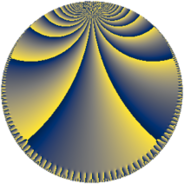# Properties

 Label 384.10.oLevel $384$ Weight $10$ Character orbit 384.o Rep. character $\chi_{384}(47,\cdot)$ Character field $\Q(\zeta_{8})$ Dimension $568$ Sturm bound $640$

# Related objects

## Defining parameters

 Level: $$N$$ $$=$$ $$384 = 2^{7} \cdot 3$$ Weight: $$k$$ $$=$$ $$10$$ Character orbit: $$[\chi]$$ $$=$$ 384.o (of order $$8$$ and degree $$4$$) Character conductor: $$\operatorname{cond}(\chi)$$ $$=$$ $$96$$ Character field: $$\Q(\zeta_{8})$$ Sturm bound: $$640$$

## Dimensions

The following table gives the dimensions of various subspaces of $$M_{10}(384, [\chi])$$.

Total New Old
Modular forms 2336 584 1752
Cusp forms 2272 568 1704
Eisenstein series 64 16 48

## Trace form

 $$568 q + 4 q^{3} + 8 q^{7} - 4 q^{9} + O(q^{10})$$ $$568 q + 4 q^{3} + 8 q^{7} - 4 q^{9} - 8 q^{13} + 8 q^{15} + 8 q^{19} - 4 q^{21} - 8 q^{25} - 12650084 q^{27} - 8 q^{33} - 8 q^{37} + 72274204 q^{39} + 8 q^{43} - 4 q^{45} + 78736 q^{51} - 140108824 q^{55} - 4 q^{57} - 180242216 q^{61} + 805675976 q^{67} - 4 q^{69} - 8 q^{73} - 7812496 q^{75} + 2269128688 q^{79} - 15625008 q^{85} + 2350951628 q^{87} + 1091566808 q^{91} + 78728 q^{93} - 16 q^{97} + 1556825028 q^{99} + O(q^{100})$$

## Decomposition of $$S_{10}^{\mathrm{new}}(384, [\chi])$$ into newform subspaces

The newforms in this space have not yet been added to the LMFDB.

## Decomposition of $$S_{10}^{\mathrm{old}}(384, [\chi])$$ into lower level spaces

$$S_{10}^{\mathrm{old}}(384, [\chi]) \cong$$ $$S_{10}^{\mathrm{new}}(96, [\chi])$$$$^{\oplus 3}$$